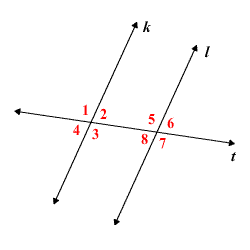# Alternate Interior Angle Theorem

The Alternate Interior Angles Theorem states that, when two parallel lines are cut by a transversal , the resulting alternate interior angles are congruent .

So, in the figure below, if $k\parallel l$ , then $\angle 2\cong \angle 8$ and $\angle 3\cong \angle 5$ .Proof.

Since $k\parallel l$ , by the Corresponding Angles Postulate ,

$\angle 1\cong \angle 5$ .

Therefore, by the definition of congruent angles ,

$m\angle 1=m\angle 5$ .

Since $\angle 1$ and $\angle 2$ form a linear pair , they are supplementary , so

$m\angle 1+m\angle 2=180°$ .

Also, $\angle 5$ and $\angle 8$ are supplementary, so

$m\angle 5+m\angle 8=180°$ .

Substituting $m\angle 1$ for $m\angle 5$ , we get

$m\angle 1+m\angle 8=180°$ .

Subtracting $m\angle 1$ from both sides, we have

$\begin{array}{l}m\angle 8=180°-m\angle 1\\ \text{\hspace{0.17em}}\text{\hspace{0.17em}}\text{\hspace{0.17em}}\text{\hspace{0.17em}}\text{\hspace{0.17em}}\text{\hspace{0.17em}}\text{\hspace{0.17em}}\text{\hspace{0.17em}}\text{\hspace{0.17em}}\text{\hspace{0.17em}}\text{\hspace{0.17em}}=m\angle 2\end{array}$ .

Therefore, $\angle 2\cong \angle 8$ .

You can prove that $\angle 3\cong \angle 5$ using the same method.

The converse of this theorem is also true; that is, if two lines $k$ and $l$ are cut by a transversal so that the alternate interior angles are congruent, then $k\parallel l$ .